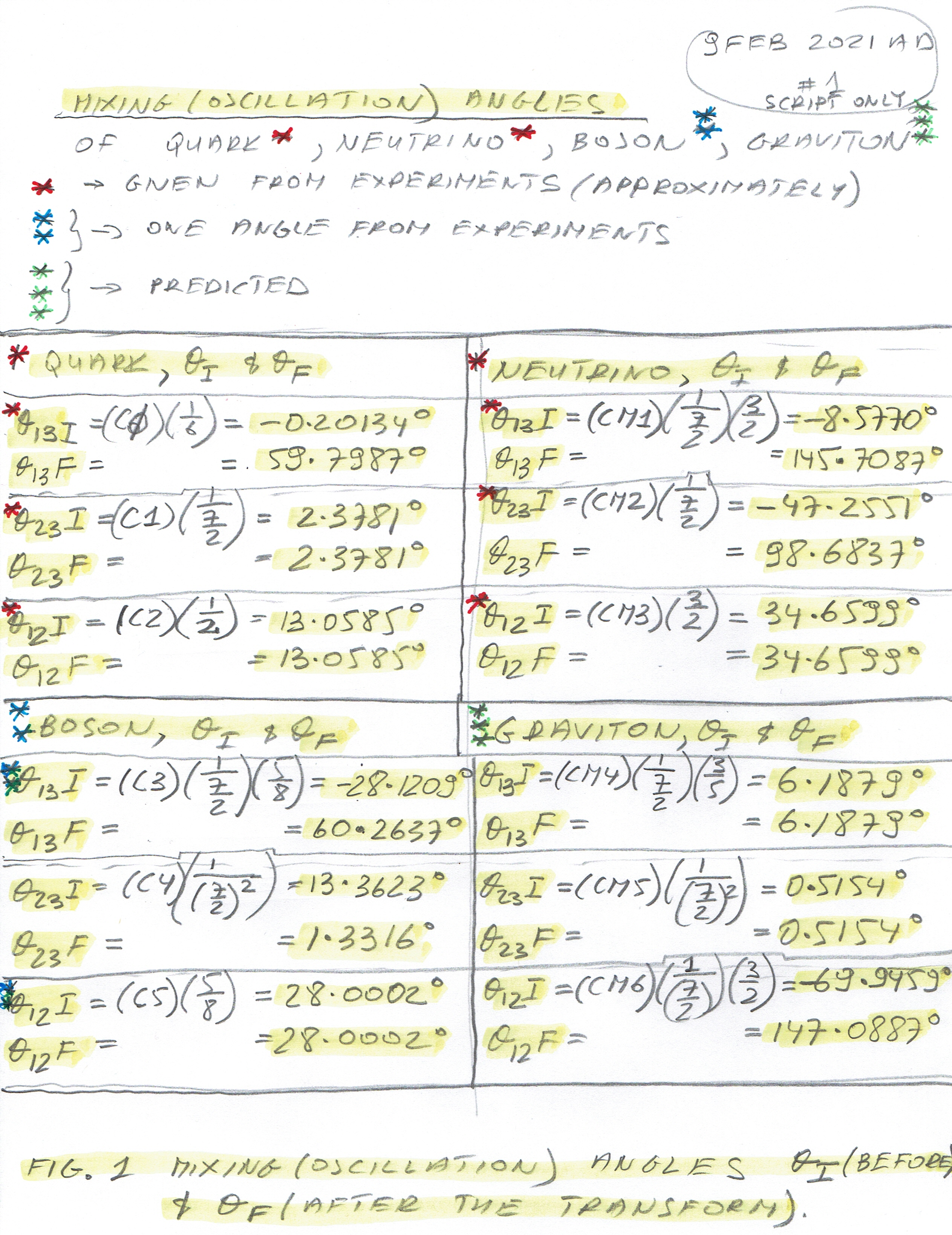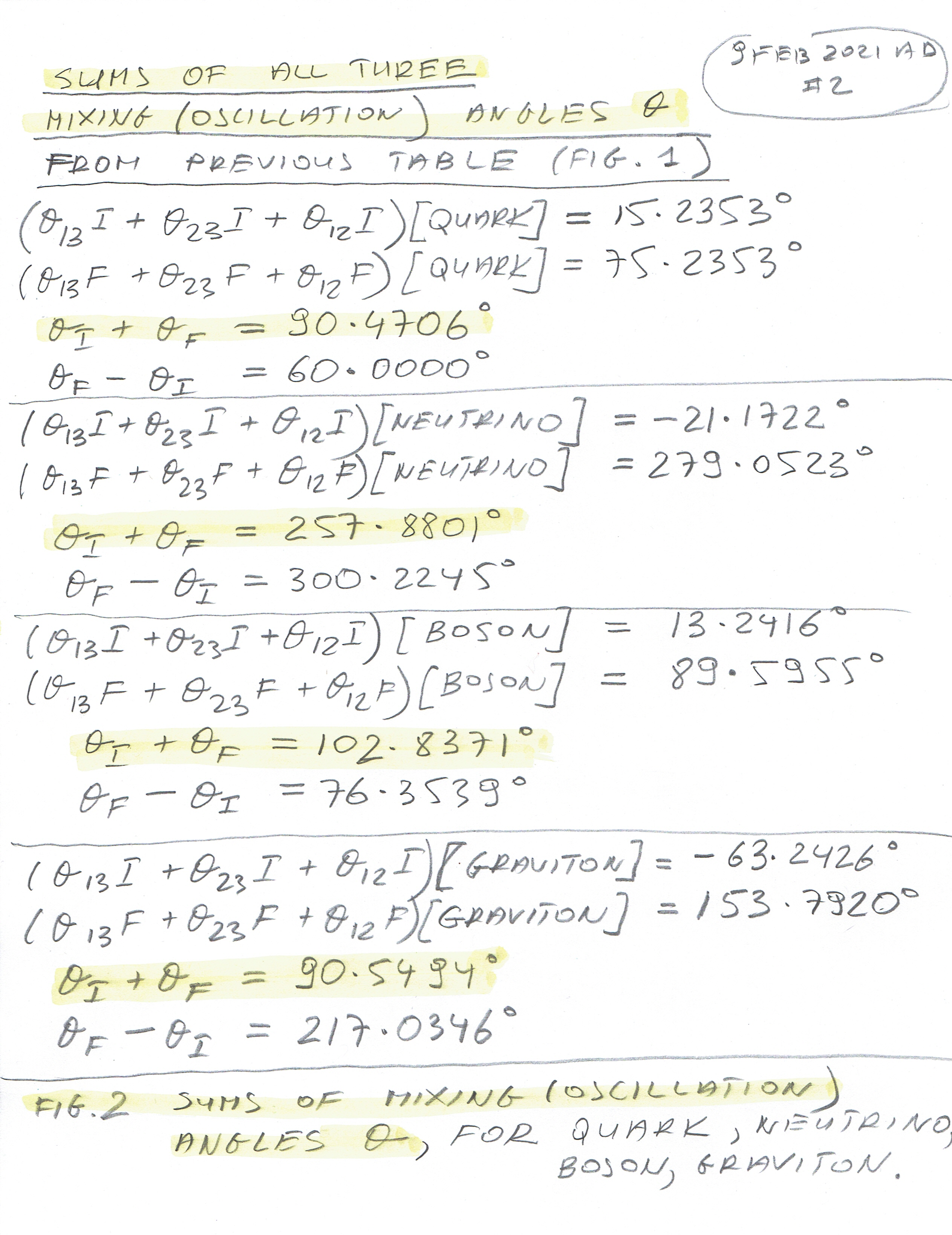St Matthias (1st Century)

This is a very easy article - it contains simple sums of all three Theta Angles: Theta(13), Theta(23) and Theta(12) from before and after the Transformation of the Angles into the Positive Real Plane.

So, we have three angles Theta in agreement with experimental data (Quark and Neutrino and one angle for Boson) and these angles after the transform, i.e. two triplets of angles.

And, of course, we have the total sum of all these six angles. These sums are extremely important since there is a strict correlation between the Sum of Mixing (Oscillation) Angles Theta and CP Violating Phase Angles Delta for all four elements (Quark, Neutrino, Bosn and Graviton) and also for the next four elements of the Universe (and Quantum as well) i.e. Space, Time, Membrane Internal and Membrane External.

In Figure 1 we have Mixing (Oscillation) Angles Theta I = Theta Initial - before the Transform and Theta F = Theta Final - after the Transform. These Angles are added together and the results are in Figure 2.

Here is Figure 1- Mixing (Oscillation) Angles Theta Initial and Theta Final.Those sums are shown in Figure 2 - "Sums of Mixing (Oscillation) Angles Theta for Quark, Neutrino, Boson and Graviton":You can read in detail in any of the following four articles (links below) how to obtain these angles and more.

75. Revisited: The Exact Value of the CO Violating Phase Angle Delta of Quark

72. Revisited: The Exact Value of the CP Violating Phase Angle Delta of Neutrino

70. The Exact Value of the CP Violating Phase Angle Delta of Boson

80. The Exact Value of the CP Violating Phase Angle delta of Graviton.

We are slowly getting to the end of the introduction to the main theme - how to get all eight Mixing (Oscillation) Angles Theta and CP Violating Phase Angles Delta for all eight Elements. There are just Quantum Fractions and Calculation of CP Violating Phase Angles remaining. After these, we get to the core of these series of articles.